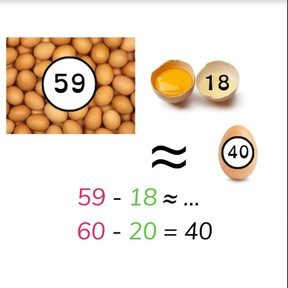Estimate the difference to 100

# Estimate the difference to 1008,000 schools use Gynzy92,000 teachers use Gynzy1,600,000 students use Gynzy

## General

Students learn to estimate the difference with numbers to 100 and learn to round numbers to the nearest ten.

2.NBT.B.5

## Relevance

Discuss with students that it is important to learn to estimate the difference so you can quickly determine about how much you have left. An example is if you have 59 eggs and 18 break- it is faster to estimate the difference.

## Introduction

The interactive whiteboard shows a grey cover. Tell the students that under this cover are ladybugs. You're going to remove the cover and you want the students to estimate how many ladybugs there are without counting them. Show the ladybugs for about 3 seconds and then cover them up again. Ask a few students to name how many ladybugs they estimate there are. There are 55 ladybugs.
Then practice rounding to the nearest ten by looking at numbers on the number line. Remind students that they are looking to see which tens number is nearest to the given number. Drag the given numbers to their nearest tens numbers.

## Development

Explain to students that when estimating the difference you start by rounding the numbers to their nearest ten. Once they are rounded, you then subtract those rounded numbers. The subtraction problem of 42 - 19 is rounded to 40 - 20. Practice this by asking students to determine which of the rounded subtraction problems given are the correct rounded subtraction problem for the subtraction problem given. Then practice coming up with the rounded subtraction problem for a given subtraction problem. Explain that you can also be asked to estimate the difference with problems with more than two numbers. When you have three or more numbers, you still follow the same steps- first round the numbers to their nearest ten, and then subtract the rounded numbers. You can also choose to add the second and third rounded numbers and take that total away from the first number. NExt students are given two realistic scenarios that involve money. The first asks students to estimate about how much money they have left, and the second asks if there is enough money. Practice rounding to the nearest ten and then subtracting.

Check that students understand estimating the difference to 100 by asking the following questions:
- Why is it useful to round numbers in a subtraction problem to estimate the difference?
- How do you round a number? What do you round 89 to? or 73?
- How do you round a number to the nearest ten?

## Guided practice

Students are given subtraction problems in which they are asked to estimate the difference. Students are also given scenarios with money, as well as given situations in which they must determine if there is enough to meet the need presented in the problem. Students are also given a few abstract subtraction problems without visual support or story context.

## Closing

Discuss with students that estimating the difference is important because it allows you to easily and quickly determine how much you have left or if you have enough. To close ask students to estimate the difference in a problem involving money and then ask the students to stand or sit to show their answer. The interactive whiteboard has three problems. Read these aloud and ask students to show their answer by standing or sitting. Yes is standing, no is sitting. Check that students are able to estimate the difference by having students drag the rounded subtraction problem to the actual subtraction problem on the interactive whiteboard.

## Teaching tips

Students who have difficulty with this learning goal should start by practicing rounding to the nearest ten as well as subtracting tens. Remind students that this should be quicker than solving exactly, and that they should round and subtract, not subtract exactly and then round.

### The online teaching platform for interactive whiteboards and displays in schools

• Save time building lessons

• Manage the classroom more efficiently

• Increase student engagement